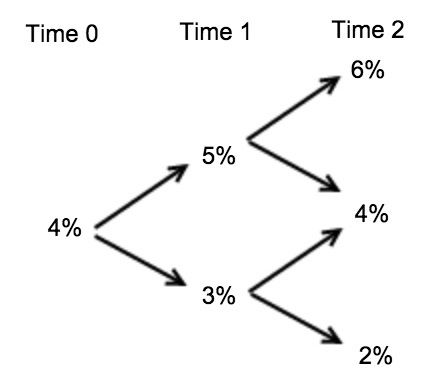### CFA Practice Question

There are 147 practice questions for this study session.

### CFA Practice Question

Given the interest rate tree below, what is the price of a three-year, annual-coupon bond with a coupon rate of 4% and par value of \$100?At time 2 we have the following values:
[104/1.06+ 104/1.06] x 0.5 + 4 = 102.1132
[104/1.04+ 104/1.04] x 0.5 + 4 = 104
[104/1.02+ 104/1.02] x 0.5 + 4 = 105.9608

At time 1 we have the following values:
[102.1132/1.05+ 104/1.05] x 0.5 + 4 = 102.1491
[104/1.03+ 105.9608/1.03] x 0.5 + 4 = 105.9227

At time 0: [102.1491/1.04+ 105.9227/1.04] x 0.5 + 4 = 100.0345